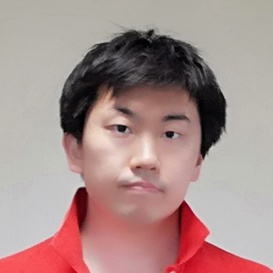atcoder

# # Problem

## # Input

$N M$
$x_1 y_1$
$x_2 y_2$
...
$x_M y_M$

• $N$ - The number of people
• $M$ - The number of relationship
• $x_i y_i$ - $x_i$ and $y_i$ know each other

## # Output

The maximum size of possible groups.
A group consists of members who know each other directly.

# # Explanation

It can be solved with bit exhaustive search as $N$ is enough small.
There are 2 choices for each member.
In case you choose to add a member to a group, you have to confirm that the member knows each existing member from the group directly.
If you reprecent the relationship as a graph, it's easy to answer this question.
The only thing you have to do is check if a edge between 2 exists.

By the way, this problem is an instance of Clique problem.

the clique problem is the computational problem of finding cliques (subsets of vertices, all adjacent to each other, also called complete subgraphs) in a graph. It has several different formulations depending on which cliques, and what information about the cliques, should be found.

# # Time complexity

$O(2^N)$

# # Solution

#define MAX_N 12
#define MAX_M (MAX_N * (MAX_N-1) / 2)

Int N, M;
Int G[MAX_N][MAX_N];

void input() {
Int x, y;
cin >> N >> M;
loop(x,0,MAX_N) {
loop(y,x + 1,MAX_N) {
G[x][y] = G[y][x] = 0;
}
}
loop(m,0,M) {
cin >> x >> y;
x--, y--;
G[x][y] = G[y][x] = 1;
}
}

Int dfs(Int n, vector<Int> members) {
if (n >= N) return members.size();
Int max_ = -1;
// Skip the member
max_ = max(max_, dfs(n + 1, members));
// Add the member if possible
// shouldAdd <=> n is connected to all the existing members
for (auto m: members) {
if (G[m][n] == 0 || G[n][m] == 0) shouldAdd = false;
}
members.push_back(n);
if (shouldAdd) max_ = max(max_, dfs(n + 1, members));
return max_;
}

void solve() {
vector<Int> members;
cout << dfs(0, members) << endl;
}

int main(void) {
input();
solve();
return 0;
}Remote freelancer. A web and mobile application enginner.
Traveling around the world based on East Asia.
I'm looking forward to your job offers from all over the world!

Offer jobs or contact me!